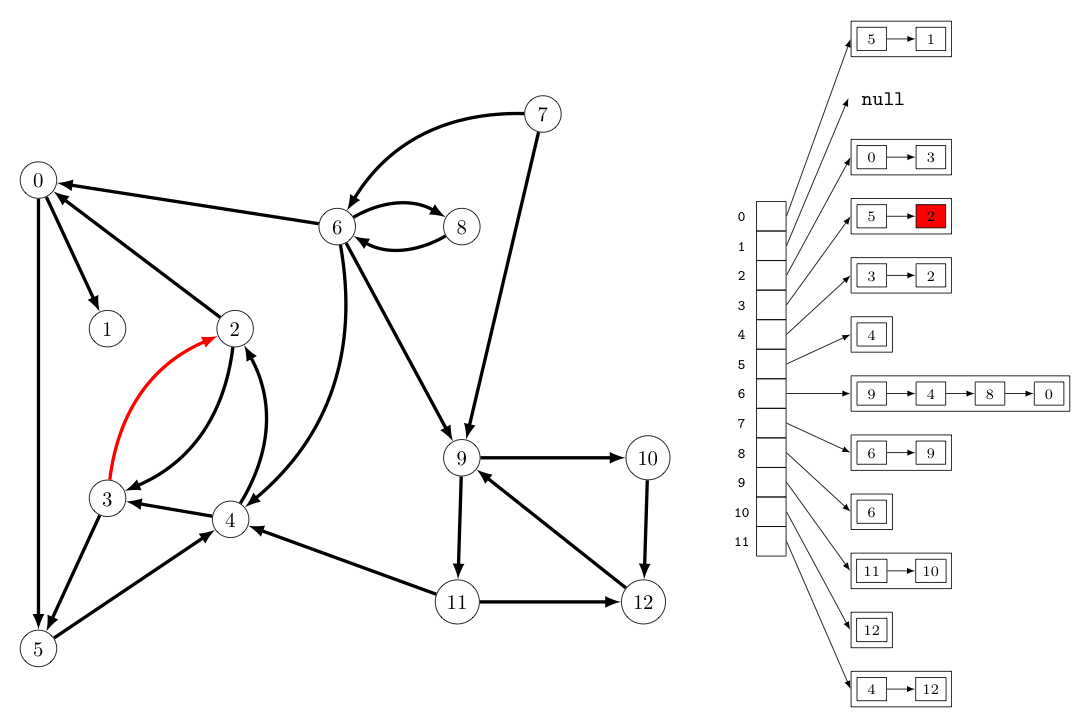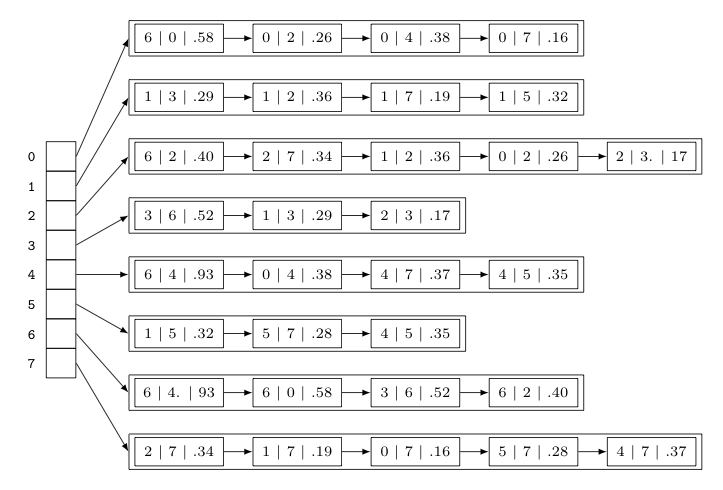## Directed and Edge-Weighted Graphs

### Directed Graphs (i.e., Digraphs)

In some cases, one finds it natural to associate each connection with a direction -- such as a graph that describes traffic flow on a network of one-way roads. Here the edges are the roads themselves, while the vertices are the intersections and/or junctions between these roads. When each connection in a graph has a direction, we call the graph a directed graph, or digraph, for short.

The directed edges of a digraph are thus defined by ordered pairs of vertices (as opposed to unordered pairs of vertices in an undirected graph) and represented with arrows in visual representations of digraphs, as shown below. Note that vertices of a digraph can now count the number of directed edges flowing away from them, known as the out degree, and the number of directed edges flowing towards them, known as the in degree. Also -- just as a graph can have paths and cycles -- a digraph has directed paths and directed cycles, except that in both of these, all of the adjacent edges must "flow" in the same direction.The following table shows some contexts in which the use of digraphs might be helpful, noting what plays the role of the vertices and directed edges in each:

Context Vertex Directed Edge
transportation street intersection one-way street
food web species predator-prey relationship
financial bank transaction
cell phone person placed call
infectious disease person infection
game board position/state legal move
research journal article citation
object graph object reference
inheritance hierarchy class extends

As with undirected graphs, the typical means for representing a digraph is an adjacency list. The only real difference is that now the list for each vertex $v$ contains only those vertices $u$ where there is a directed edge from $v$ to $u$. As such, we no longer have each edge showing up twice in the adjacency list. The graph below provides an example.The time and space complexity is similar to undirected graphs as well, except now -- given that edges directed towards any vertex $v$ don't add to the bag of edges maintained at adj[v] -- the limit on the time to either check if there is an edge between vertices $v$ and $w$ or to iterate over the vertices associated with $v$ are now both linear in terms of the out degree of $v$, as seen in the table below.

representation space add edge edge between $v$ and $w$? iterate over vertices pointing from $v$
list of edges $E$ $1$ $E$ $E$
adjacency matrix $V^2$ $1$* $1$ $V$
adjacency lists $E+V$ $1$ $\textrm{out degree}(v)$ $\textrm{out degree}(v)$
* this representation does not allow for multiple edges

### Edge-Weighted Graphs

In other cases, it is more natural to associate with each connection some numerical "weight". Such a graph is called an edge-weighted graph. An example is shown below. Note, the weights involved may represent the lengths of the edges, but they need not always do so. As an example, when describing a neural network, some neurons are more strongly linked than others. If the vertices of the graph represent the individual neurons, and edges represent connections between pairs of neurons, than the weight of an edge might measure the strength of the connection between two associated neurons.With regard to representation, we still employ adjacency lists -- but with a structural tweak. We need to store the edge weights, so rather than making the lists associated with each vertex $v$ a list of integers corresponding to the vertices adjacent to $v$, we make them lists of edges incident to $v$. Making a separate Edge class will be convenient to this end. In this way the adjacency lists have a structure similar to what is shown below (which represents the edge-weighted graph immediately above).### Edge-Weighted Digraphs

Still other graphs might require both edges with both weights and direction. Not surprisingly, such graphs are called edge-weighted digraphs. Appealing to economics this time for an example, note that a graph could be used to describe the flow of money between a group of individuals in a given time period. Using vertices to represent the individuals involved, two vertices could be connected if any money flowed from one to the other. The net amount of money that changed hands provides a weight for the edges of such a graph, and the direction of the connection could point towards the vertex that saw a net gain from the associated transactions.